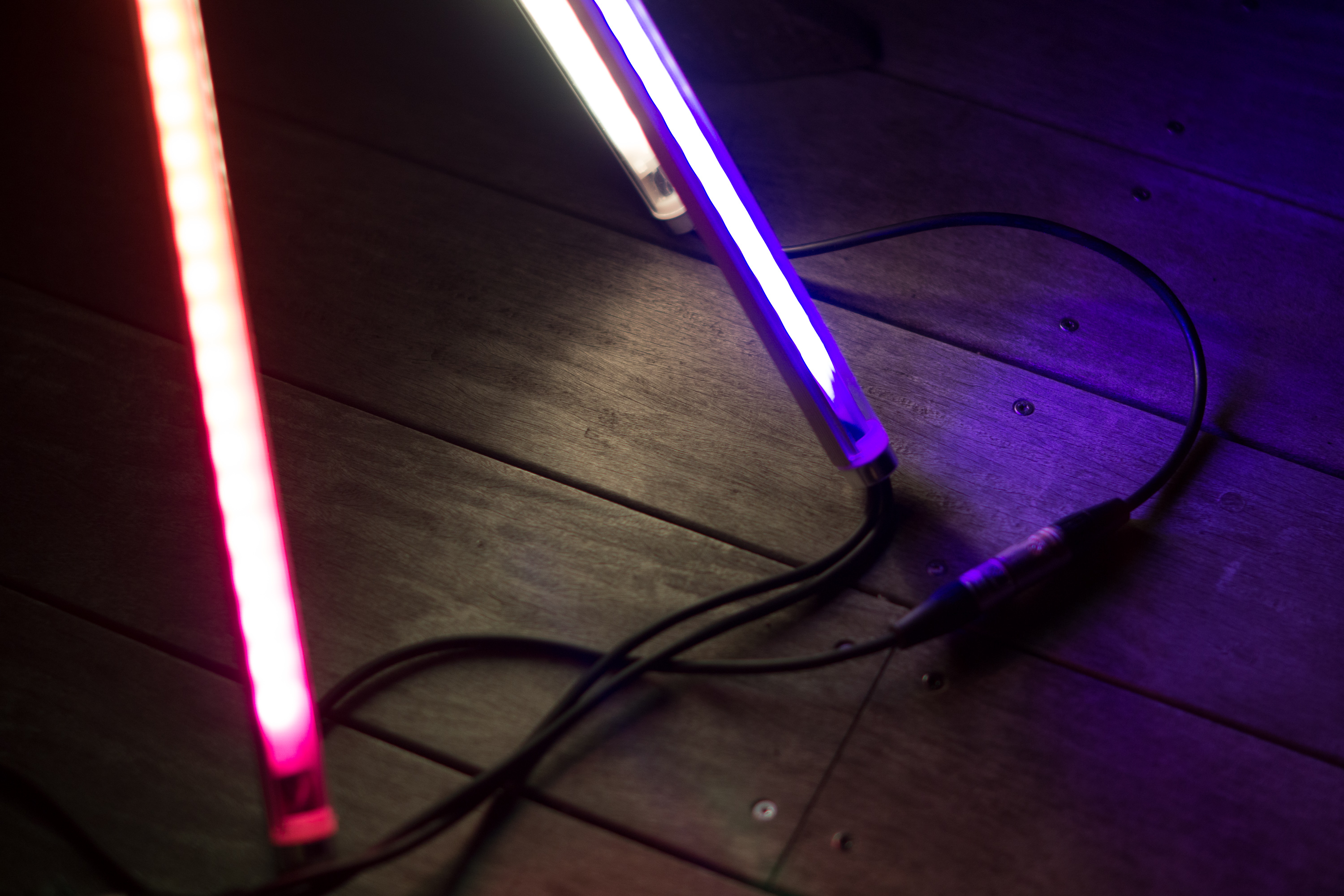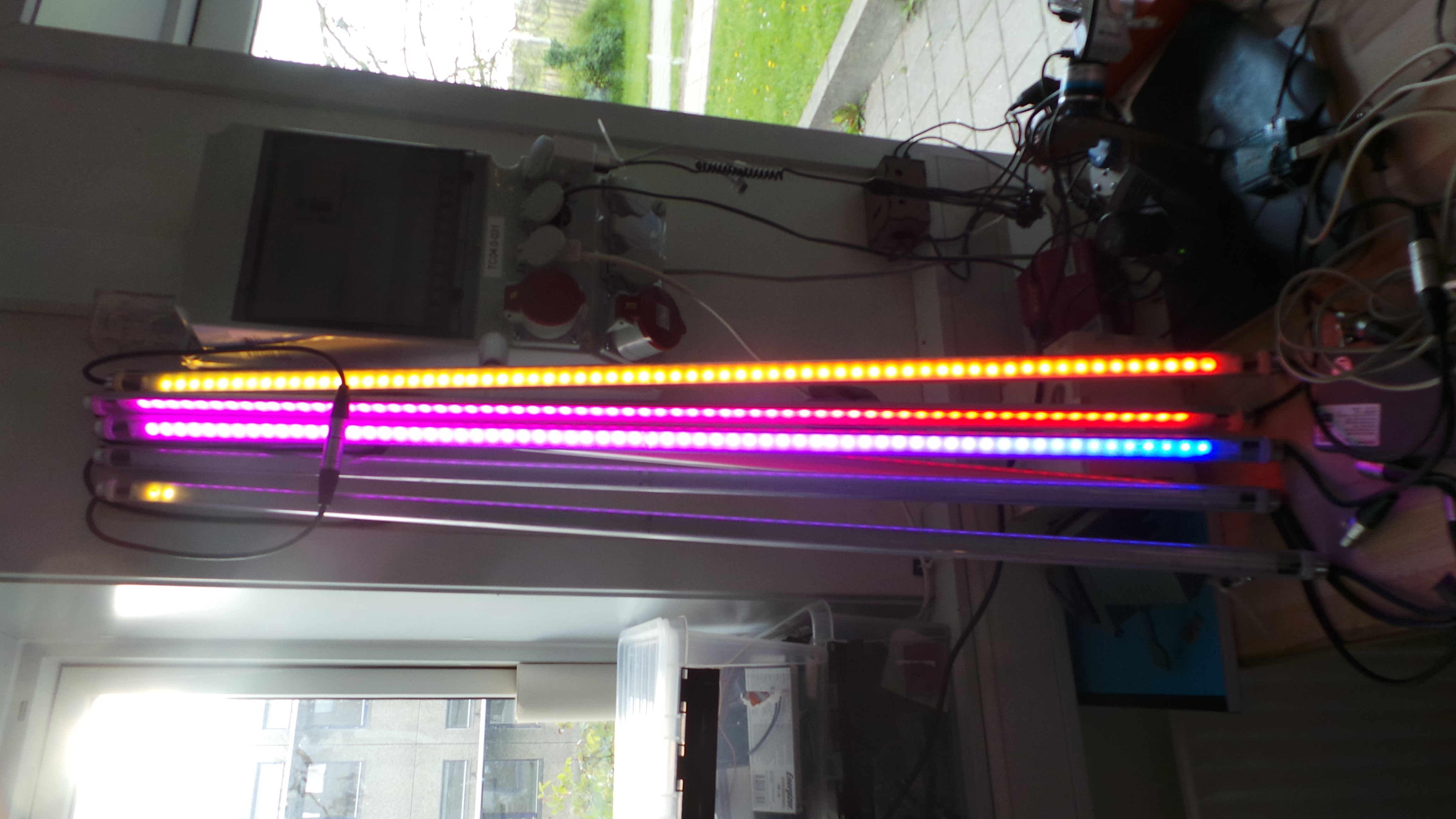Published: May 11, 2015, Edited by: Mads Hobye

# Neosticks: Turning neopixels into lightmodulesThe potential of using neopixels as moular modules for light installations is an obvious thought. The challange is to create the physical enclosure that makes them highly configurable and still robust enough to be experimented with.

Small video demonstrating a rainbow pattern (code below):

This version is made out of a discarded LED light tubes for fluorescent light fixtures. Mads Hobye gutted the original content and replaced it with neopixels instead. Each has an xlr female/male connector to connect multiple tubes in serial.The same tubes with the new content:A few of the tubes has both the male and female xlr connector in the same end - enabling star shaped formations:In this case the there is a wire for the data connector running behind the led strip back to the female connector.

The neopixel strip is mounted on two pieces of acrylic plate laser cut to fit inside the tubes:The Arduino testmodule wiring looks like this:#### Power limitations to be aware of

The light modules requires a significant amount of power. Powering a long string of modules wron the end point might not be possible through the rather thin xlr cables. To solve this it is neccessary to make a T connector power injector with a female/male xlr that can be embedded for after three led modules. Further it is recommended to have cable running behind the led strip inside each module. The cable should provide power. This way the power is not only running through the thin tracings on the strip itself.

#### Test code (Using Adafruit neopixel library):

``````#include <Adafruit_NeoPixel.h>

#define PIN 7
#define NUM_PIXELS 200

// Parameter 1 = number of pixels in strip
// Parameter 2 = Arduino pin number (most are valid)
// Parameter 3 = pixel type flags, add together as needed:
//   NEO_KHZ800  800 KHz bitstream (most NeoPixel products w/WS2812 LEDs)
//   NEO_KHZ400  400 KHz (classic 'v1' (not v2) FLORA pixels, WS2811 drivers)
//   NEO_GRB     Pixels are wired for GRB bitstream (most NeoPixel products)
//   NEO_RGB     Pixels are wired for RGB bitstream (v1 FLORA pixels, not v2)

// IMPORTANT: To reduce NeoPixel burnout risk, add 1000 uF capacitor across
// pixel power leads, add 300 - 500 Ohm resistor on first pixel's data input
// and minimize distance between Arduino and first pixel.  Avoid connecting
// on a live circuit...if you must, connect GND first.

float h = 0;
float offset = 0;

void setup() {
strip.begin();
strip.show(); // Initialize all pixels to 'off'
}

void loop() {
h = 0;
for(int i =0; i < NUM_PIXELS; i ++)
{
h = h + 1.0f;

strip.setPixelColor(i, HSLtoRGB( round(h + offset)% 360,100,50));
}
offset = offset + 10.0f;
strip.show();

}

// This function converts the "color" object to the equivalent RGB values of
// the hue, saturation, and luminance passed as h, s, and l respectively

uint32_t HSLtoRGB(float h, const float s, float l)
{
int tmpR,tmpG,tmpB;
unsigned int r = 0;
unsigned int g = 0;
unsigned int b = 0;

float L = ((float)l)/100;
float S = ((float)s)/100;
float H = ((float)h)/360;

if(s == 0)
{
r = l;
g = l;
b = l;
}
else
{
float temp1 = 0;
if(L < .50)
{
temp1 = L*(1 + S);
}
else
{
temp1 = L + S - (L*S);
}

float temp2 = 2*L - temp1;

float temp3 = 0;
for(int i = 0 ; i < 3 ; i++)
{
switch(i)
{
case 0: // red
{
temp3 = H + .33333f;
if(temp3 > 1)
temp3 -= 1;
HSLtoRGB_Subfunction(r,temp1,temp2,temp3);
break;
}
case 1: // green
{
temp3 = H;
HSLtoRGB_Subfunction(g,temp1,temp2,temp3);
break;
}
case 2: // blue
{
temp3 = H - .33333f;
if(temp3 < 0)
temp3 += 1;
HSLtoRGB_Subfunction(b,temp1,temp2,temp3);
break;
}
default:
{

}
}
}
}
tmpR = round((((float)r)/100.0)*255.0);
tmpG= round((((float)g)/100.0)*255.0);
tmpB = round((((float)b)/100.0)*255.0);
return strip.Color(tmpR,tmpG,tmpB);

}
static void HSLtoRGB_Subfunction(unsigned int& c, const float& temp1, const float& temp2, const float& temp3)
{
if((temp3 * 6) < 1)
c = (unsigned int)((temp2 + (temp1 - temp2)*6*temp3)*100);
else
if((temp3 * 2) < 1)
c = (unsigned int)(temp1*100);
else
if((temp3 * 3) < 2)
c = (unsigned int)((temp2 + (temp1 - temp2)*(.66666 - temp3)*6)*100);
else
c = (unsigned int)(temp2*100);
return;
}
``````